Statistics and Data Analysis - New Features in Maple 2019 - Maplesoft# Statistics and Data Analysis

Least Trimmed Squares Regression

The LeastTrimmedSquares command computes least trimmed squares regression for some data.

 >In this example, we have 1000 data points. There is a single independent variable, x, with values uniformly distributed between 0 and 10. The dependent variable is a linear function of the independent variable plus some additive noise, y=5 x + 10 + noise, where the noise is from a probability distribution known to have severe outliers - the Cauchy distribution, with location parameter 0 and scale parameter 5.

 >>>Here we see all data points:

 >Linear least squares regression will be severely affected by the outliers.

 >>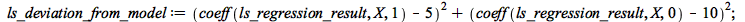Least trimmed squares regression gets much closer to the true line without noise.

 >>The result is even better if we include 900 out of the 1000 points, instead of the default of a little over 500.

 >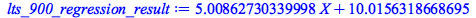>The other robust regression method, implemented in the RepeatedMedianEstimator procedure, also gets a good result.

 >>In order to visualize these results, we show the same point plot as before, including the four regression lines. The three regression lines from robust methods cannot be distinguished, but the least squares method is clearly off. We zoom in on the vertical range that includes most points.

 >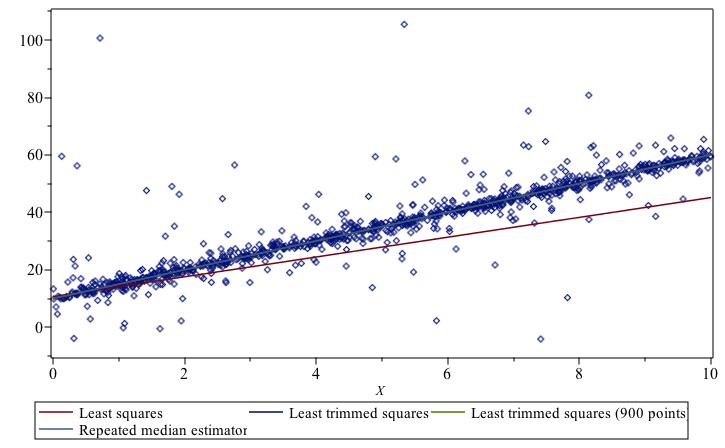Correlogram

The Correlogram command computes autocorrelations of a data set and displays the result as a column plot with dashed lines indicating the lower and upper 95% confidence bands for the normal distribution N(0,1/L), where L is the size of the sample 'X', and a caption reporting how many of the displayed columns lie outside of the bands of plus or minus 2, 3, and 4 standard deviations respectively. AutoCorrelationPlot is an alias for the Correlogram command.

 >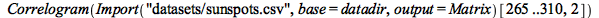Detrend

The Detrend command removes any trend from a set of data.

 >>For example, specify some data:

 >Fit a linear model to the data:

 >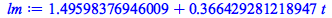It can be observed that from the plot of the data and the linear model that there is some upward trend. The Detrend command removes any trend from the data.

 >This can be observed in the following plot:

 >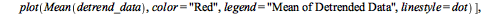Detrend has also been added as an option to several routines in SignalProcessing including SignalPlot, Periodogram, and Spectrogram.

Difference

The Difference command computes lagged differences between elements in a data set.

 >Define some data:

 >>Display of Data Structures

The default display of data structures such as rtables (matrices, vectors, and arrays) as well as data frames and data series has been changed. Previously a summary would be shown for the data structure, but now the display shows the header of the data (by default the first 10 rows and 10 columns) and the size of the data structure.

 > Matrix(20,20,rand(1..10));DataFrames and DataSeries

Several commands have been updated to support DataFrames and DataSeries, including remove, select and selectremove. Other new commands such as Detrend and Difference also support DataFrames and DataSeries.

Biplot

The Biplot command has a new option, components, which specifies the principal components used in the biplot.

DataSummary, FivePointSummary, FrequencyTable

The DataSummary, FivePointSummary, and FrequencyTable commands have a new option, tableweights, which specifies the relative column widths in the displayed embedded table.